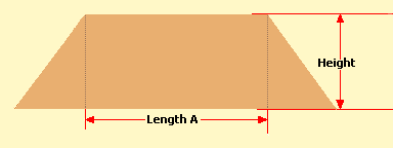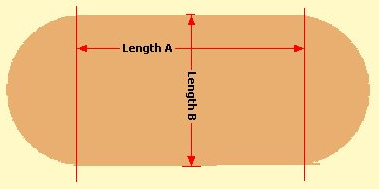Spike's Calculators

# Calculate the Volume of a Pile in Cubic YardsEstimate the volume of an excavated pile of material in cubic yards.If you look at the second image, the footprint, length A is not the overall length.
If you subtract that from the total length and add both ends, you end up with a cone that has a diameter that is equal to length B.

##### The calculation is done in two parts.
The volume of the cone is calculated as:
```V = 1/3 * π * R² *  H
V = the volume in cubic feet
π = 3.14159265
R = length B divided by 2
H = Height```
The volume of the center prism:
```0.5  *  A * B * H
V = the volume in cubic feet
A = length A
B = length B
H = height```
The two results are added together for a cubic foot value and converted to cubic yards by dividing the cubic feet by 27.
Enter the height of the pile and the base length of the pile in feet.

### Volume of a Pile/ Cubic Yards

Length A ft
Length B ft
Height ft

#### Results:

 Cubic Feet ft³ Cubic Yard yd³

#### The Calculator

1. Enter the length A in feet
2. Enter the length B in feet
3. Enter the height of the pile in feet

#### The Results

1. The volume of the pile in cubic feet
2. The volume of the pile in cubic yards